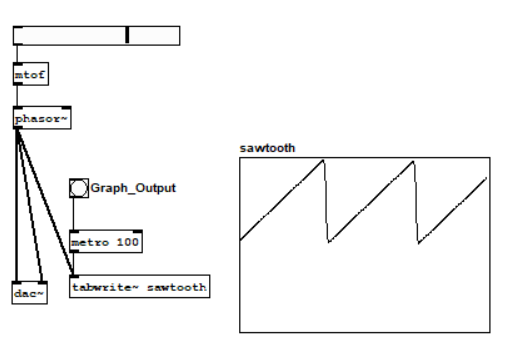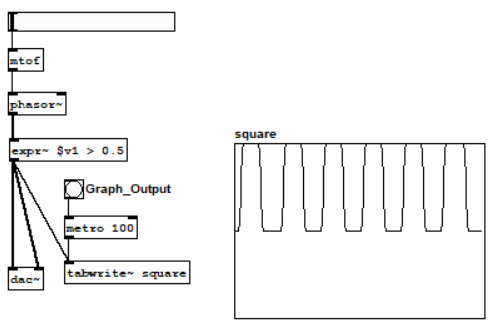I am continuing to follow along with the FLOSS Manuals Pure Data, and have gotten up to making Oscillators. As I needed to do slightly more research than just reading the manual to follow along with the tutorial (I also needed to watch this video to figure out the steps), I shall list the steps.

## What are Oscillators?

Oscillators are used to generate the signals that are combined to make output sounds in computer music. PureData expects output of generators to have values $$x \in [-1,1]$$. The oscillators that I made, as instructed by the tutorial, were sine, sawtooth, and square wave oscillators.

I need to do more research about exactly what oscillators are, but this shall happen later.

## How do you make an oscillator in Pure Data?

### General

The general steps for making an oscillator in Pure Data are as follows:

1. Give a numeric value.
2. Convert MIDI notes to frequency in Hertz.
3. Make the numeric value input to the MIDI notes to frequency in Hertz converter.
4. Define the oscillator’s waveform.
5. Make the frequency in Hertz input into the waveform.
6. Create a line out to the soundcard.
7. Put the waveform’s output as input to the soundcart output.
8. Write out a table of the waveform’s output.
9. Put the waveform as input to the table.
10. Make a frequency of sampling.
11. Put the frequency of sampling as input to the table.
12. Make a graph.
13. Make an indicator that connects the table to a graph.
14. Connect the graph indicator to the frequency sampling as an input.

Technically, we needn’t graph to make a sound, but it’s nice to be able to see what’s happening.

### Sine Wave

The steps followed to make a sine wave oscillator, with variable inputs, were as follows:

1. Put > HSlider
2. Put > Object > mtof
3. Connect 1 to 2, 1 as input to 2.
4. Put > Object > osc~
5. Connect 2 to 4, 2 as input to 4.
6. Put > Object > dac~
7. Connect 4 to 6, 4 as input to both o f 6’s input boxes
8. Put > Object > tabwrite~ sine
9. Connect 4 to 8, 4 as input to 8.
10. Put > Object > metro 100
11. Connect 10 to 8, 10 as input to 8
12. Put > Array > name > sine
13. Put > Bang > label > Graph Output
14. Connect 14 to 10

That’s it! Your code should look like the following. (Yes, puredata is a picture based language.)### Sawtooth Wave Oscillator

This is really similar. Follow the directions above, but change the following steps:

• Step 4: Put > Object > phasor~
• Step 8: Put > Object > tabwrite~ sawtooth
• Step 12: Put > Array > name > sawtooth

You should have code that looks like:### Square Wave Oscillator

Also really similar. Follow the directions above, but change the following steps:

• Step 4: Put > Object > phasor~

• Step 4a: (continued) Put > Object > expr~ \$v1 > 0.5

• Step 8: Put > Object > tabwrite~ square

• Step 12: Put > Array > name > square

You should have code that looks like: